# 11.10 The circuit shown in Fig. P11.10 uses a 12-V ac source to deliver power to...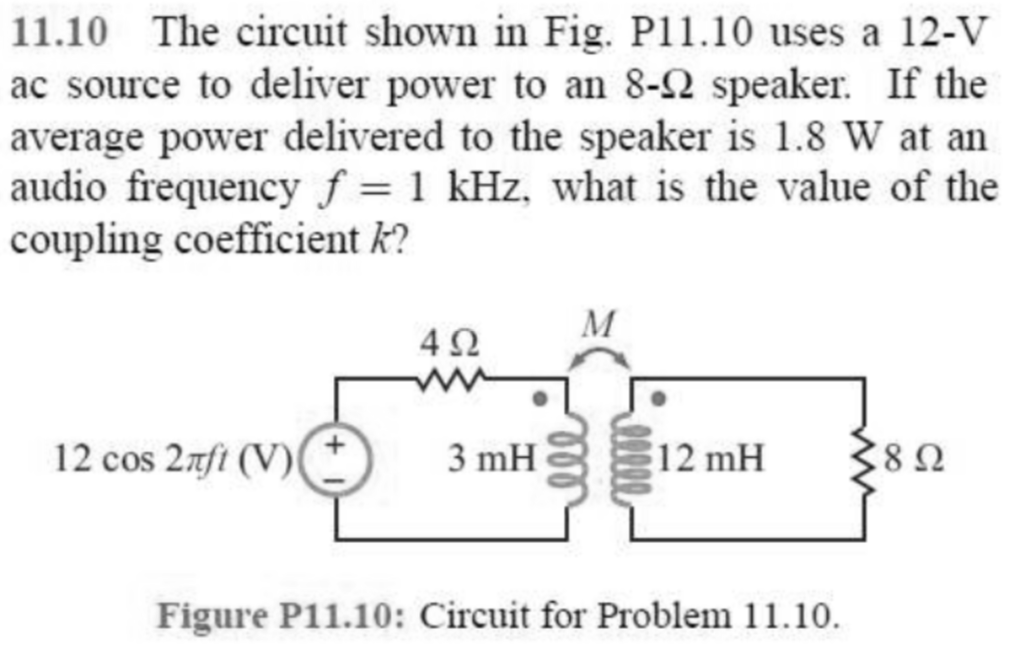11.10 The circuit shown in Fig. P11.10 uses a 12-V ac source to deliver power to an 8-Ω speaker. If the average power delivered to the speaker is 1.8 W at an audio frequency f= 1 kHz, what is the value of the coupling coefficient k? 4Ω 12 cos 2z/t (V) 3mH 12 mH 8Ω Figure P11.10: Circuit for Problem 11.10.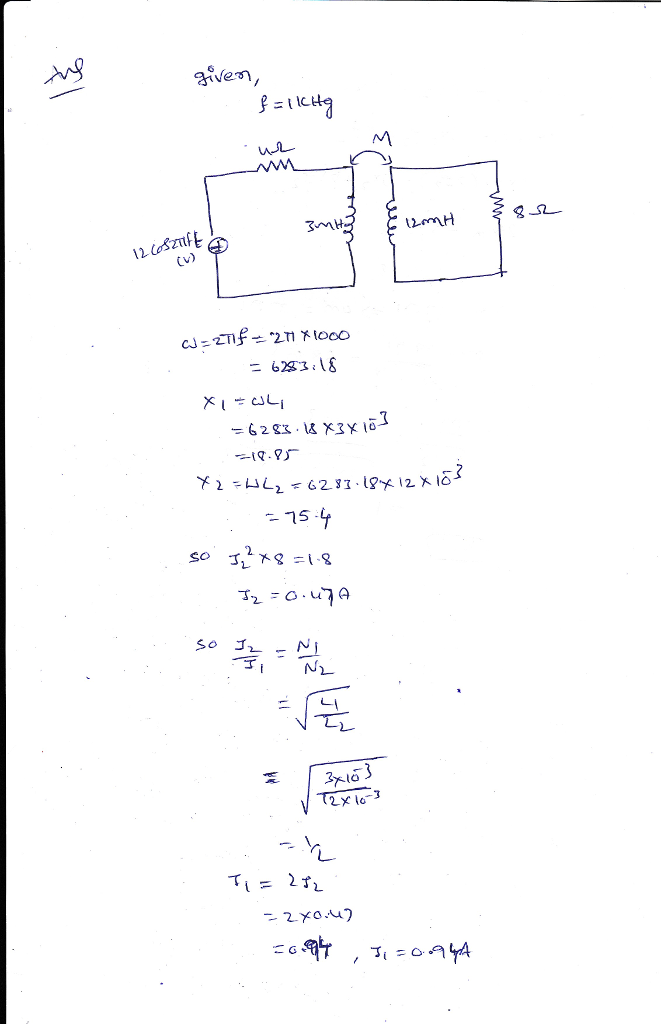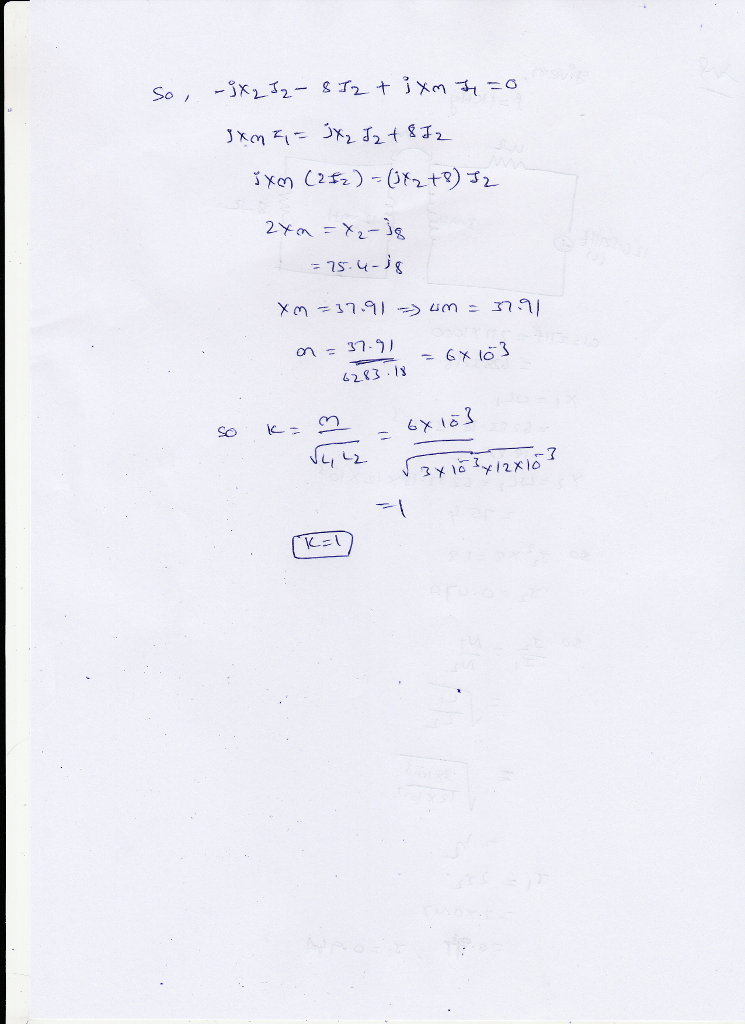#### Earn Coin

Coins can be redeemed for fabulous gifts.

Similar Homework Help Questions
• ### An audio amplifier, represented by the AC source and the resistor R in the figure below,...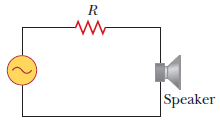An audio amplifier, represented by the AC source and the resistor R in the figure below, delivers alternating voltages at audio frequencies to the speaker. If the source puts out an alternating voltage of 12.0 V (rms), the resistance R is 8.20 Ω, and the speaker is equivalent to a resistance of 12.4 Ω, what is the time-averaged power delivered to the speaker?

• ### 20Ω 30 Ω j20Ω Fig. 1.7 In the circuit of Fig. 1.8, find the RMS phasor...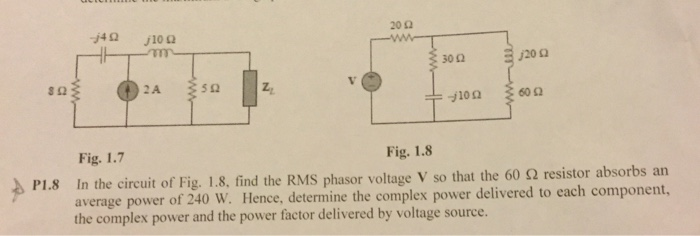20Ω 30 Ω j20Ω Fig. 1.7 In the circuit of Fig. 1.8, find the RMS phasor voltage V so that the 60 Ω resistor absorbs an average power of 240 W. Hence, determine the complex power delivered to each component, the complex power and the power factor delivered by voltage source. Fig. 1.8 2) P1.8

• ### Question 35 (6 points) 35. For the circuit in the figure shown, (a) what is the...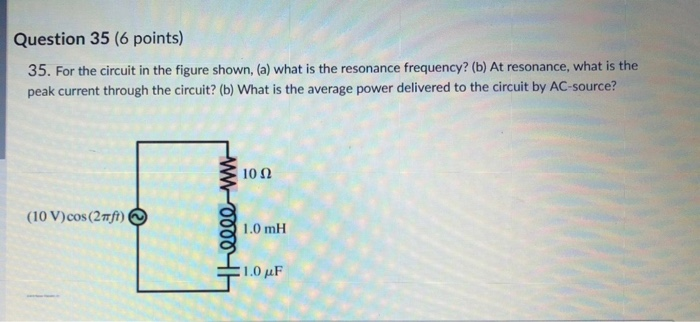Question 35 (6 points) 35. For the circuit in the figure shown, (a) what is the resonance frequency? (b) At resonance, what is the peak current through the circuit? (b) What is the average power delivered to the circuit by AC-source? 10 Ω (10 V)cos ( 21) 1.0 mH Shelll-w 1.Ο μF

• ### 9) The voltage V in the frequency-domain circuit shown in Fig. P10.18 is 240/0°V (rms) a)...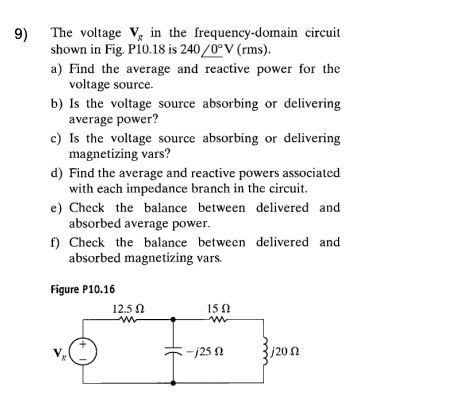9) The voltage V in the frequency-domain circuit shown in Fig. P10.18 is 240/0°V (rms) a) Find the average and reactive power for the voltage source. average power? magnetizing vars? with each impedance branch in the circuit. absorbed average power. absorbed magnetizing vars. b) Is the voltage source absorbing or delivering c) Is the voltage source absorbing or delivering d) Find the average and reactive powers associated e) Check the balance between delivered and f) Check the balance between delivered...

• ### Exercise 31 A series ac circuit contains a 350 - resistor, a 20.0 - mH inductor,...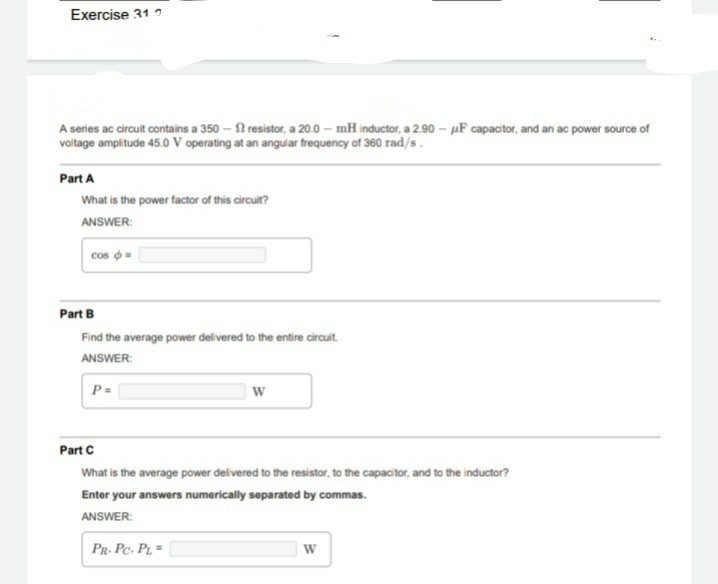Exercise 31 A series ac circuit contains a 350 - resistor, a 20.0 - mH inductor, a 2.90 - uF capacitor, and an ac power source of voltage amplitude 45.0 V operating at an angular frequency of 360 rad/s. Part A What is the power factor of this circuit? ANSWER: cos - Part B Find the average power delivered to the entire circuit ANSWER: P- w Part C What is the average power delivered to the resistor, to the capacitor,...

• ### A series R-L-C circuit is connected to a 110 Hz ac source that has Vrms =...

A series R-L-C circuit is connected to a 110 Hz ac source that has Vrms = 80.0 V . The circuit has a resistance of 65.0 Ω and an impedance of 104 Ω at this frequency. What average power is delivered to the circuit by the source?

• ### 35. For the circuit in the figure shown, (a) what is the resonance frequency? (b) At...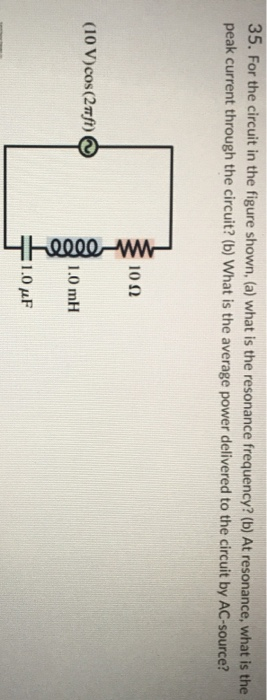35. For the circuit in the figure shown, (a) what is the resonance frequency? (b) At resonance, what is the peak current through the circuit? (b) What is the average power delivered to the circuit by AC-source? 1012 (10 V)cos (2 ft) Yhelle-w 1.0 mH 1.0 pF

• ### An AC generator provides power for the circuit shown below. The generator voltage is described by...

An AC generator provides power for the circuit shown below. The generator voltage is described by E(t)=Emaxsin(ωt)E(t)=Emaxsin⁡(ωt) where E=E= 120 V. Here, L=L= 640 mH, C=C= 160 μμF, R=R= 90 ΩΩ, and ω=ω= 40 rad/sec. 1) What is the phase angle between the generator and the current? ϕ=ϕ= -55 degrees ϕ=ϕ= 1.1 degrees ϕ=ϕ= 64 degrees ϕ=ϕ= -16 degrees ϕ=ϕ= -0.99 degrees Your submissions: A Submitted: Wednesday, July 29 at 1:02 PM Feedback: Feedback will be available after 11:59 PM...

• ### An AC circuit has a voltage source 11.0cos(wt) V. There is also a 550. Ω resistor...

An AC circuit has a voltage source 11.0cos(wt) V. There is also a 550. Ω resistor and a 1.10 nF capacitor in the circuit. What is the value of the peak voltages VR and VC if the emf frequency is 4.20 kHz? VR= Vc=

• ### Power in an AC Circuit 1 2 3 4 5 6 A circuit is constructed with...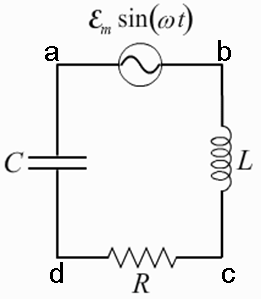Power in an AC Circuit 1 2 3 4 5 6 A circuit is constructed with an AC generator, a resistor, capacitor and inductor as shown. The generator voltage varies in time as ε =Va - Vb = εmsinωt, where εm = 120 V and ω = 314 radians/second. The inductance L = 283 mH. The values for the capacitance C and the resistance R are unkown. What is known is that the current in the circuit leads the voltage...

Free Homework App